## How to calculate Z-Scores in Tableau

by Emily Dowling

What is a Z-Score?

In statistics, the z-score is the relationship of a data point to the mean of a group of data points. Z-Scores are measured by the number of standard deviations from the mean.

The chart below displays the distribution of the sum of profits within a specific subcategory for a specific State, (each individual data point represents a State). The distance from any of these individual data points to the mean is the z-score.

If a data point is greater than the mean then the z-score will be positive; if the data point is less than the mean, the z-score will be negative.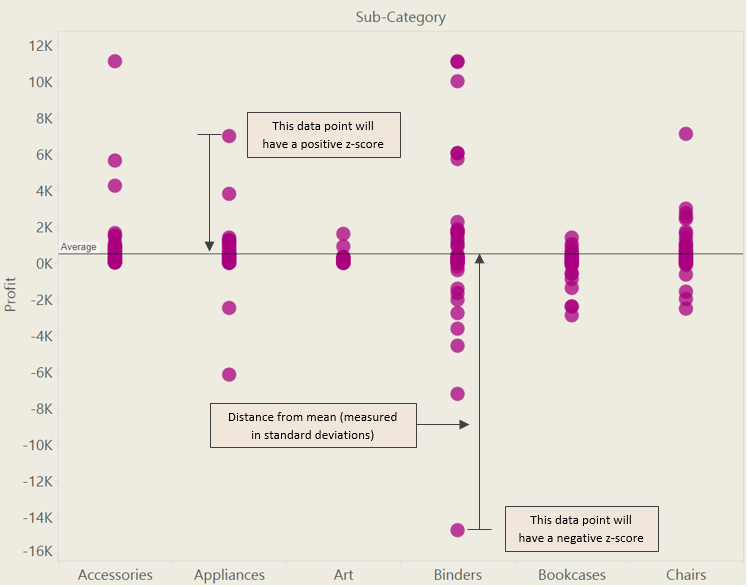Why are Z-Scores Useful?

Z-Scores are useful because they can help you understand the distribution of your data. They can tell you whether a particular data point is equal to, above or below the mean, and how far it is from the mean. They are also useful as a comparison for different groups of data from different normal distributions.

If data is normally distributed, typically 99% of the data z-scores will fall between -3 and +3, 95% between -2 and +2, and 68% between -1 and +1.

How to calculate Z-Scores

To calculate the z-score you subtract the mean from and individual raw score (where your data point sits on the y axis) then dividing the difference by the standard deviation.

I calculated the z-scores for my data points by first building a simple view in Tableau. Using the Tableau Superstore Sales sample dataset, I put Subcategory on columns, Sum of Profit on rows and then State onto detail.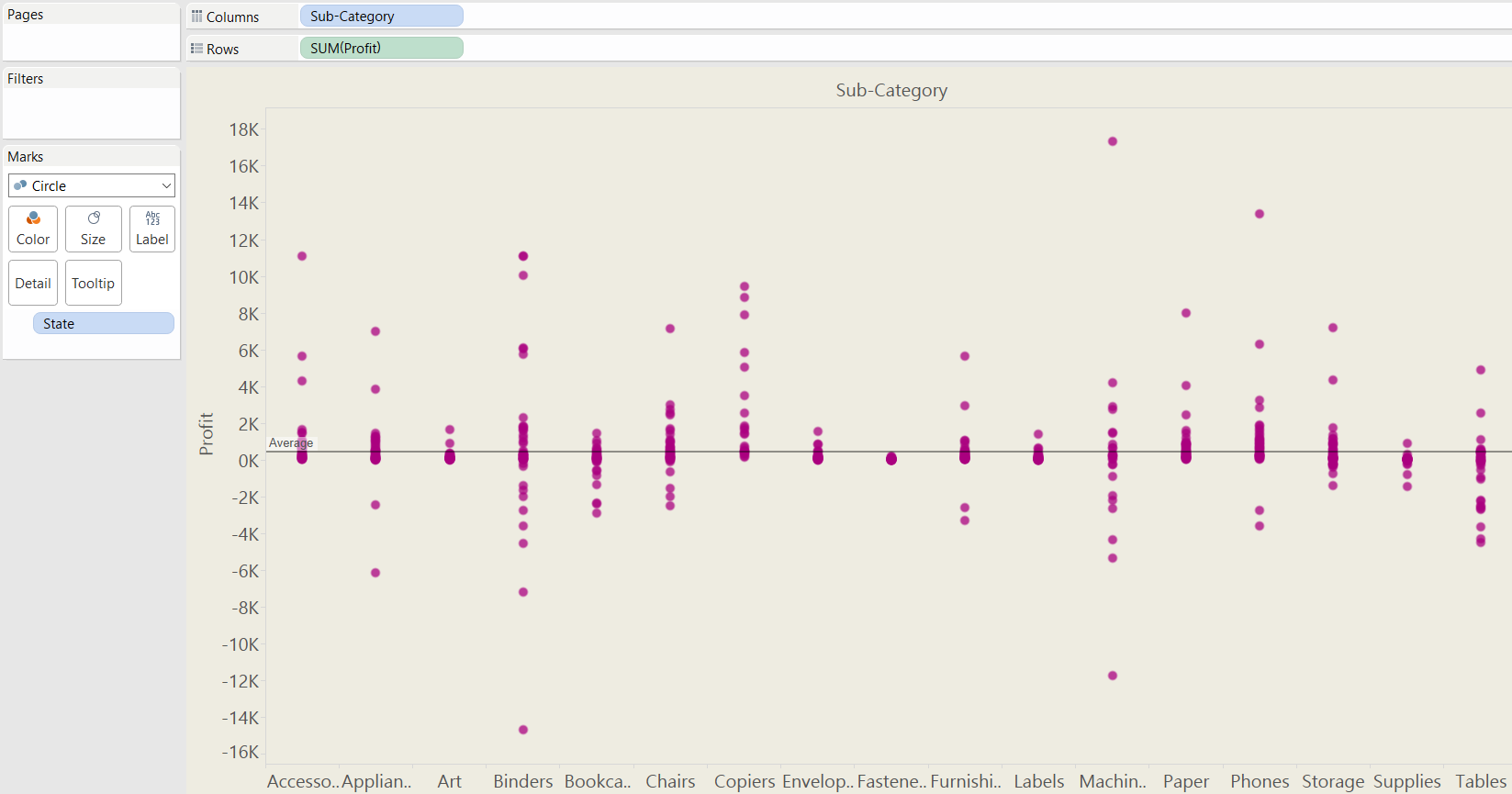The basic equation to calculate a z-score is…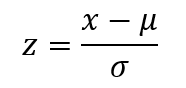…where X is the SUM(profit), Sigma () is the standard deviation and Mu () is the mean.

Each of the data points in the view is a sum of profit (x) and therefore you need to find the average of all the different points (States) within the scope of the window, so to translate this to Tableau, you need to use the window average function. This is also the case to find the standard deviation.

Therefore, the calculation for Mu (), the mean, is:

WINDOW_AVG(SUM([Profit]))

And the calculation for Sigma (), the standard deviation, is:

WINDOW_STDEV(SUM([Profit]))

So the final calculation is: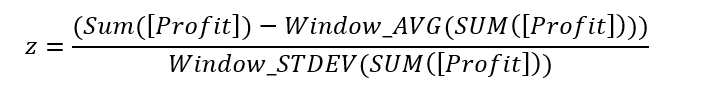Therefore, the calculation in Tableau-speak is:

(SUM([Profit])-WINDOW_AVG(SUM([Profit]))) / WINDOW_STDEV(SUM([Profit]))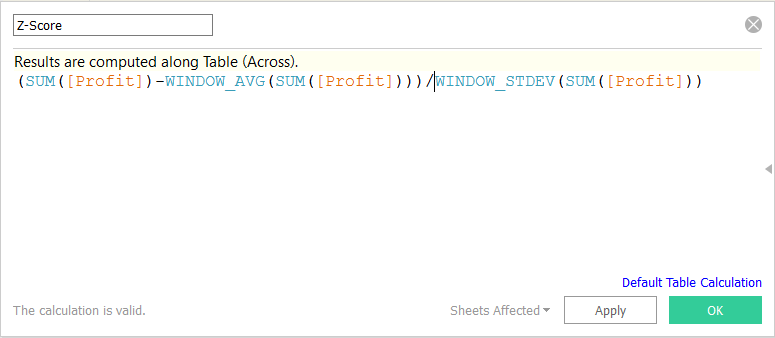Because the window average is used (which is a table calculation) this means that the Profit has to be aggregated, hence SUM. It also means that the calculation needs to be computed by something, in this case you need to compute the table calculation using State.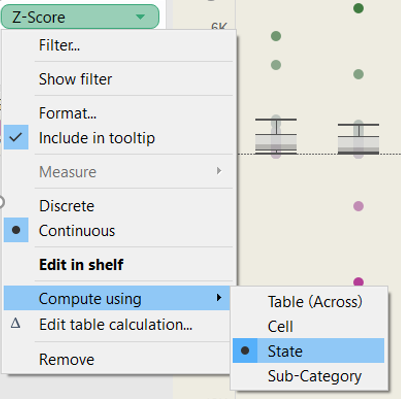Once you have created the calculated field in Tableau, you can drag the field onto labels, or the tooltips to see the calculated z-score for all of the data points. You can also drag the calculated field onto colour and set a diverging colour scheme to differentiate the positive z-scores from the negative z-scores and to see the distance from the mean (i.e. the gradient).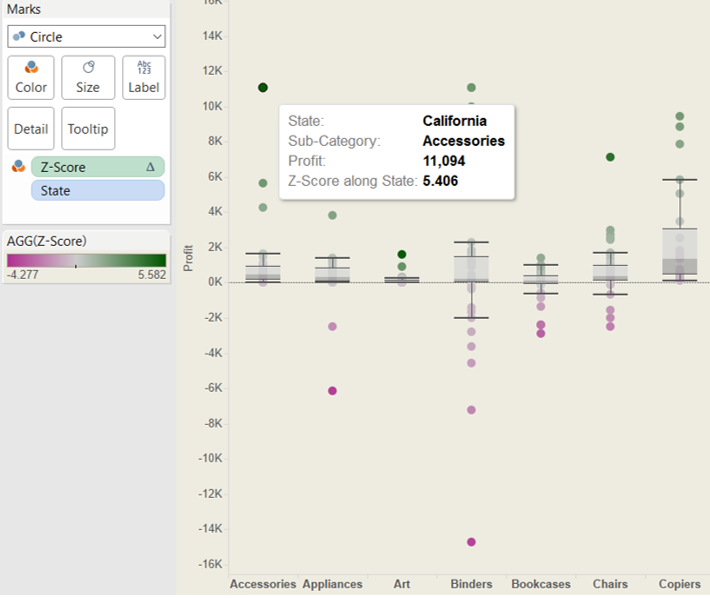Emily Dowling

Fri 02 Sep 2016

Mon 08 Aug 2016

Fri 05 Aug 2016

Thu 07 Jul 2016